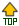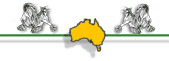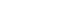Site Index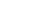# How to Choose Your Witch Name

Names are very important!

Great thought and your inner feelings will play the major roles while choosing your witch name.  This can sometimes be confusing and frustrating.  But I have placed here a guideline to use while searching for that most personal name in hopes that it will give more meaning and understanding to the process.  Your Birth Number and Name Number should be the same.

NUMEROLOGICALLY!!!

Sometimes numerology is forgotten in choosing a name.  Below you will find a system of numerology that is most commonly used.

## Numerological Formula for Names

1.  How to find your Birth Number.

Add all the numbers of your birthdate.
Example:  March 10, 1954 =  3+1+0+1+9+5+4 = 23

Bring down the final two digits (23) and add together.
Example:  2+3 = 5

is the Birth Number.

2.  Now to find out if the name you have choosen is appropriate for you.

Alphabet and number equation.

 1 2 3 4 5 6 7 8 9 A B C D E F G H I J K L M N O P Q R S T U V W X Y Z
Now by using the chart above calulate the name you have choosen by adding the letters and final sum.

Example:    name = DIANA,  D = 4,  I = 9,  A = 1,  N = 5,  A = 1

4 + 9 + 1 + 5 + 1 = 20

Bring down the sum and add:

2 + 0 = 2        2 is the Name number.

Now your birth number is 5 so you will need to add a letter equivilate to 3 (C, L, U) or you could add 2 letters, (one equivulate to 1 (A,J,S) and one equivulate to 2 ( B, K, T).

Example:    Dilana = 4 + 9 + 3 + 1 + 5 + 1 = 23,    2 + 3 = 5

Diakana = 4 + 9 + 1 + 2 + 1 + 5 + 1 = 23,    2 + 3 = 5

If you don't like any of the names that the are created to equal 5, try another name.

I hope this will help in you quest to find the name that is suitable for you.

Brightest of Blessings!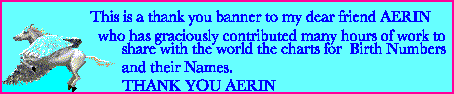Here you will find charts of names corresponding to birth numbers.

 Female Names, Birth # 1 and # 2 Female Names, Birth # 3 and # 4 Female Names, Birth # 5 and # 6 Female Names, Birth # 7, # 8 and # 9

 Male Names, Birth # 1 and # 2 Male Names, Birth # 3 and # 4 Male Names, Birth # 5 and # 6 Male Names, Birth # 7, # 8 and # 9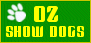E-mail Us to report a broken link!